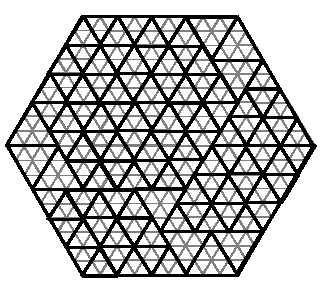Problem 1069. -- The Bermuda Triangle

## POJ_1069: The Bermuda Triangle

Time Limit: 2000 MS  Memory Limit: 33 MB   64bit IO Format: %I64d
Submitted: 0  Accepted: 0
[Submit][Status][Web Board]

## Description

People in the hidden region of the Bermuda Triangle make everything they need in triangular shapes. One day, someone decided to break the rule and bake a hexagonally shaped cake. But as usual, he has to serve the cake in triangular pieces. The pieces are equilateral triangles but in different sizes for different people. He can use as many triangles as needed to cut the cake into pieces, such that nothing remains from the cake. For example, the following figure shows one way that a hexagon with side 9 can be cut into triangles with side 2 and 3. (The cake is cut along the thick lines, thin lines are drawn to show the sizes).Input is a hexagon and triangle types (specified by the length of their sides) and the goal is to decide if the hexagon can be completely divided by the given triangle types.

## Input

The first line of the input file contains a single integer t (1 <= t <= 10), the number of test cases, followed by the input data for each test case. Each test case consists of a single line, containing s (1 <= s <= 25), the length of the hexagon's side, followed by n, the number of triangle types (1 <= n <= 10), followed by n integers representing the length of each triangle type's side (between 1 and 25, inclusive).

## Output

There should be one output line per test case containing either YES or NO depending on whether the hexagon can be completely divided by the given triangle types.

```3
5 2 2 3
7 2 3 2
13 2 2 3
```

## Sample Output

```NO
NO
YES
```

[Submit][Status][Web Board]